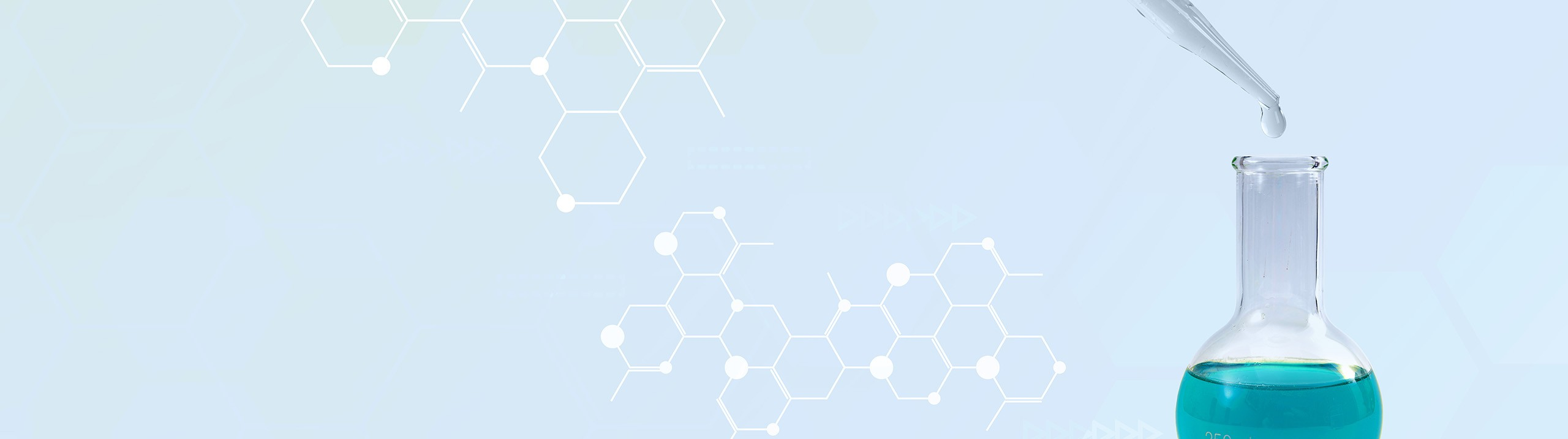## Analytical Chemistry

Learn the toughest concepts covered in your Analytical Chemistry class with step-by-step video tutorials and practice problems.

1. Chemical Measurements

# Volumetric Analysis

The concentration of solutions can be expressed in terms beyond molarity and molality.

Percent Composition
1
concept

## Percent Composition Terms1m
Play a video:
Now in addition to polarity and morality and mole fraction, we can discuss the concentrations of solutions in various other ways. Now here we have weight percent, volume percent and weight volume percent. Now when it comes to weight percent, or sometimes called mass percent or percent by mass, we say that mass of our solute divided by the mass of the solution times 100 gives us this new expression used for discussing the concentrations of solutions. In addition to this we have volume percent now, whereas mass percent uses grams over graham's volume percent typically uses milliliters over milliliters. So we have milliliters of our solute divided by volume or milliliters of our solution times 100. And then finally we have a mixture of the two which is weight over volume percent. This is typically used within the medical field. In this case we're going to have the weight of our salute on top, usually in grams divided by the volume of our solution on the bottom, which will be in middle leaders or centimeters cubed. They're the same thing on the bottom and then you multiply that by 100 introducing these new types of expressions for the concentrations of solutions. Opens the door to different types of word problems that can still give us questions dealing with polarity and morality. So based on everything we've learned of this thus far, let's take a look at example one and see how best to approach that question to get the answer that we want

Weight percent represents the mass composition of solute within a solution.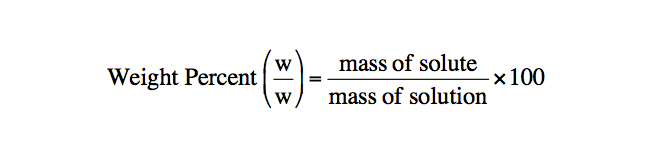Volume Percent represents the volume composition of solute within a solution.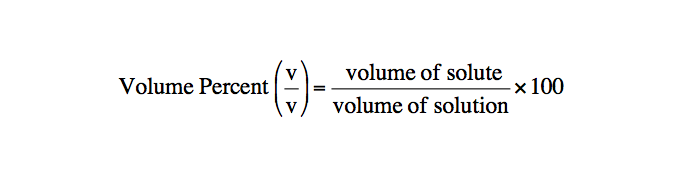Weight/Volume Percent combines aspects of both weight percent and volume percent.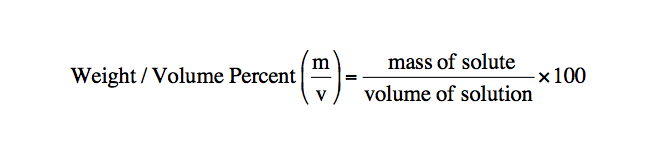2
example

## Weight Percent of Sulfuric Acid2m
Play a video:
So here it says a solution is prepared by dissolving 18.83 g of sulfuric acid. Enough water to make 250 ml of solution. If the density the solution is 1.1094 g per milliliter, What is the weight percent of sulfuric acid in the solution? Were also given the molecular weight of sulfuric acid is being 98.86 g per mole here. They want us to find the weight percent of sulfuric acid. So here we're gonna say that weight percent or mass percent would equal the grams of our sulfuric acid divided by grams of our solution. And that would be times 100. Next we look and see what the given information is. The given information that we have is we have 18.83 g of sulfuric acid. We have the volume of our solution as 250 MS of solution. And were given the density of our solution as being 1.1094 g of solution for everyone. Middle liter of solution realize here that right off the bat, we already know how many grams of sulfuric acid we have. So we can plug that in right away. So we have 18.83 g of sulfuric acid on top. We want grams of solution on the bottom. So if we multiply the volume of the density together, the volume of my solution, cancel out. And I'm gonna have my grams of solution at the end. When we multiply those two numbers. That gives me 277.35 g of solution. And then just simply multiply that by a 100 And we'll have our percentage at the end. So here we're gonna have 6.78926 here, this has four sig figs. This technically has two sig figs. And this here has 56 figs. So we're gonna go with two significant figures at the end. So that's gonna give me 6.8% for my sulfuric acid solution. So based on what we've seen in this first example, attempt to do the next example, remember the key to any of these questions is to first figure out what they're asking us to find. Then on the opposite side, write down all the given information. What you need to find can always be located somewhere within the given information. You just may have to manipulate things or isolate, isolate things to get into that one last unit that you require. So, good luck guys again, if you get stuck, don't worry, just come back and see how I approach this example too
3
example

## Parts per million of Lead5m
Play a video:
Percent Composition Calculations
4
example

## Percent Composition4m
Play a video:
in the following example, we're told 8.13% aluminum sulfate solution has a measured density of 1.235 g per milliliter, calculate the molar concentration of sulfate ions in the solution. Now realize here that we don't want the polarity of the aluminum sulfate solution, which just want the polarity of the sulfate ions themselves. So here we're looking for polarity of sulfate ions. So that would just be the moles of sulfate ions divided by the leaders of solution. What information are we given while we're told that we have 8.13% of aluminum sulfate solution so that 8.13%. What does that really mean? Well, that means that we have 8.13g of aluminum sulfate for every 100 g of solution. We're also told that the density of the solution is 1.35 g of solution For every one ml of solution. In addition we're given the mass of aluminum sulfate. But with these types of calculations we need that we can write that down to well incorporated within our calculations. Now, these are the two pieces of information that we're being given. And with this we have to figure out the polarity of sulfate ions. So what we're gonna do here is we're gonna start out by bringing down the mass percent of aluminum sulfate solution. We have 8.13 g of aluminum sulfate And that's over 100 g of solution. What we're gonna do first is going to convert the grams of aluminum sulfate into molds of aluminum sulfate by using the molecular mass or molecular weight they were given within the question. We want to get rid of Graham. So goes on the bottom. So we have 342.17 g of aluminum sulfate on the bottom. And then we have one mole of aluminum sulfate on top. So what happens here is this cancels out with this? The reason we have to figure out the moles of aluminum sulfate, is that once we have the molds of the entire compound, we can do a multiple comparison to find the molds of just sulfate ions. At this point we're going to say that for every one mole of the entire aluminum sulfate on compound, there are exactly three moles of sulfate ions. So that's three moles of sulfate ions. So the moles cancel out At this point, we have moles of sulfate ion over grams of solution. We're not done yet because we need leaders of solution on the bottom. Now, how do I get rid of those grams of solution? Well, we have the density of our solution there, we're going to bring that down. We want to cancel out these grams of solution here on the bottom. So we're gonna have the grams of solution of the density on top And then one middle leader of solution on the bottom. So grams of solution cancel out Now I have middle leaders of solution on the bottom. I want to cancel out those mls. So I'm gonna put 1000 mls of solution on top And then one liter of solution on the bottom so that at the end what do we have? We have exactly moles of sulfate on top leaders of solution on the bottom. That will give us the polarity of sulfate ions within the solution. So that gives me 0.880312 molar sulfate ions. Now based on the information given we have 366 here, 466 here. So we'll go with three significant figures for our answer. So that gives me 30.880 moller sulfate ions. As our final answer. Now that you've seen this approach. Look to see if you can figure out how to set up example to this one is vastly different from the first one we just did. Um So if you can't approach it, don't worry, just come back and see how I tackle this um example to so attempted on your own. If you're stuck, just come back and see how I approach that same question
5
example

## Percent Composition4m
Play a video:
Alright. So here it says the density of a 33.8 solution of sodium acetate is 1.10 g per milliliter, it says a reaction requires 68.8 g of sodium acetate. Um What volume of the solution do you need if you want to use a 50% excess of sodium acetate. Alright, so this question is a bit different from what we've seen in the past. We're dealing here with an excess of of a particular compound. But before we talk about that access, let's see what they're asking us to find. They want us to find the volume of the solution. So here we're looking for the milliliters. I'm just gonna say milliliters as my default amount or units milliliters of solution. Next, we're gonna write down all the given information and from that will solve for the milliliters of solution. Alright, so we're told that we have 33.8% solution. So that means we have 33.8 g of sodium acetate Divided by 100 g of solution. We're told that we have a density of solution that's 1.10 g per meal leader. So that's 1.10 g of solution per one millimeter of solution. And we can see here that the mls of solution. I want to isolate. We can find them right here. So we know that we're gonna end with a density of solution in some way to get milliliters of solution at the end. Also they're telling us that we required 68.8 g of sodium acetate, but that we want a 50% excess of that. So what exactly does that mean? Well, in a reaction, when we're talking about theoretical yield, theoretical yield represents 100%. What we want now is we want an additional 50%. So we want 150%. But when it comes to percentages, we don't use them in our calculations that way, what we're gonna do is we're gonna divide this by 100. So that's gonna come out to 1.50. We're gonna take that 1.50 and multiply it by the 68.8 g of sodium acetate. That will give us our 50% access that we need. So multiply it by 1.50. That gives me 103.2 g of sodium acetate. Now we know that we want to isolate mls of solution. We're gonna start off by using first grams of sodium acetate because it's the easiest to manipulate because it's just one unit. So we have 100 and 3.2 g of sodium acetate. These grams of sodium acid. Taking cancel out with these grams of sodium acetate. So we're gonna put on the bottom 33.8 g of sodium acetate And 100 g of solution on top. Now we want to cancel out those grams of solution. So take the density, we take the 1.10 g of solution. Put them on the bottom And then the one middle liter of solution on top. So grams of solution cancel out. So at the end I'll have mls of solution. This comes out to 277.569 ml of solution here. This has three significant figures, three significant figures. Three significant figures. Uh 50%. Um that has one significant figures, but we'll go with the other ones. So that gives me 2 78 mls of my solution. Okay, so that's my answer there. So we're accustomed to seeing these types of word problems. The new twist was looking at excess, realized that when we're talking about theoretical yield, that's 100%. So any excess would just be that number added to the 100%. We would divide that, that new percentage by 100 to get its decimal form and then multiply by the theoretical yield, which in this case was 68.8 g of sodium acetate. Doing that helped us to isolate the volume of my solution in the end. Now we've seen this one. Try to attempt a practice question left here on the bottom again. Don't worry if you get stuck, just come back and see how I approach that practice problem left at the bottom of the page
6
Problem

How many grams of nitric acid, HNO­3, and water are found in 53.1 g of 83.1 mass percent nitric acid?

Dilutions
7
example

## Dilution1m
Play a video: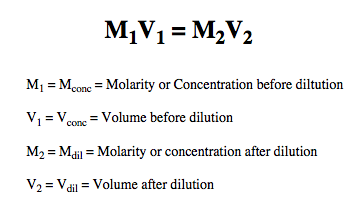8
example

## Dilutions3m
Play a video:
here. It's asking how many grams of 53.1 weight percent sodium chloride should be diluted to 2.50 liters to make 0.15 moller and a cl. Now in this question we're not dealing with M one V one equals M two V two because we only have one volume and one polarity um involved within the question what this question itself is asking? It's asking us for the grams of solution. So how many grams of solution are needed based on what they've told us. Now with this information, we're gonna put all the given information on the other side and organize it to help isolate our grams of solution. So we have 53.1 weight percent sodium chloride solution. So that means we have 53.1 g of sodium chloride Over 100 g of solution. There goes the grams of solution. We know we need to isolate at the very end of all our calculations, we're told that we have 2.50 liters and then we're given a polarity of 0.15. What does that really mean? While polarity is moles over liters. So 0.15 molar really means that I have 0.15 moles of sodium chloride Per one L of solution. So we know we need to isolate my grams of solution. We're gonna start out first with the 2.50 L because it's just one unit by itself, easier to manipulate. So we have 2.50 L. We want to cancel out leaders. So we're gonna bring down the polarity, we're gonna have the one liter of solution here on the bottom And we're gonna have .15 moles of sodium chloride here on top. Leaders cancel out. At the moment. We have moles of sodium chloride. We know that. We need to isolate these grams of solution to be able to do that. We need to cancel out these grams of sodium chloride. That means that the molds that I have right now have to change into grams. So the molecular weight that were given for sodium chloride we can use. Now We're gonna say one mole of sodium chloride on the bottom weighs 58.443 g of sodium chloride on top. So moles cancel out. Now I have grams of sodium chloride Now that I finally have those grams of NACL. I can use them to cancel out the 53.1 g of NACL. So we have 53.1 g of NACL. Here on the bottom. 100 g of solution on top. When we plug that in, that's gonna give me 41.27 33 g of solution. Now, if we look that's three significant figures. Three significant figures. Two significant figures. So at the end, we're going to say we need approximately 41g of solution to accomplish this. Now, taking a look at this example, let's see if you guys can attempt the practice problem that's left on the bottom, Come back, take a look and see how I approach that same exact question.
9
Problem

If 920 mL of water is added to 78.0 mL of a 1.28 M HBrO4 solution what is the resulting molarity?

Dilutions Calculations
10
example

## Dilutions4m
Play a video:
here we're told to calculate the density of 15.9 mls of a 49.1% by weight of acquis per cleric acid when it is diluted to 825 mls of 8250.100 moller per chlorate acid solution. All right. So, they want us to find density of this solution. So that would mean that I would need the grams of my per caloric acid solution divided by the mls or centimeters cubed of my per caloric acid solution. From the given information. We realize first that we already have the volume of my per clerk asset solution needed for the density that's found right here. They're asking me to find the density of 15.9 mls. So that right there we plug right on the bottom and that's the volume or mls of my solution. All we have to do now is figure out what my new g of solution will be after diluting uh this 49.1% by weight per Clark acid solution to these new concentration and new volume. Alright, so we're going to say that the word of when it's in between two numbers means multiply and realize here that polarity is moles over leaders. So that means if I can change these mls to leaders, I can multiply these together and isolate moles at the end. So I'm gonna take the 825 mls I'm gonna say here for everyone. Leader I have 1000 mL. Next we're gonna stay here. That 0.100 moller really means that I have 0.100 moles of per caloric acid over one liter. So leaders here cancel out again. We want grams of our solution at the end. Where are we going to get those grams of solution? Well, we're told what the percent By weight or weight percent is. That's 49.1 g of perk Lorik acid for every 100 g of solution. Here are the grams of solution. I need to isolate and to be able to isolate them. I'm gonna have to cancel out those grams of per caloric acid on top. So right now we're we have moles. Within our calculations, we're gonna have to change those moles into g. We're told that the molecular weight of per cleric acid is 100.461 g per mole. So for every one mole of per caloric acid, I have 100.461 g of per caloric acid moles cancel out. And I finally have isolated grams of perk Lorik acid. Lastly take the weight percent that were given the very beginning of the question. Take those grams and plug them here on the bottom. Take the 100 g of solution and plug him on top. When we do that, we'll isolate our grams of solution. So when we do that, we get 16.8799 g of per chlorate acid solution. Take that and plug it in 16.8799 g over 15.9 MLS When we do that, that gives me 1.06163 g/m2. If we look at all the numbers given this has three sig figs, three sig figs, three sig figs and three sig figs, which would mean at the end we want our density to also be three significant figures. So it comes out to 1.06 g per mil a leader as the density for my solution, now that we've seen that example, attempt to do this final example on this page as always if you get stuck or just want to see how I approach it. Just click over to the next video and see how we approach this question to get our desired answer.
11
example

## Dilutions5m
Play a video: